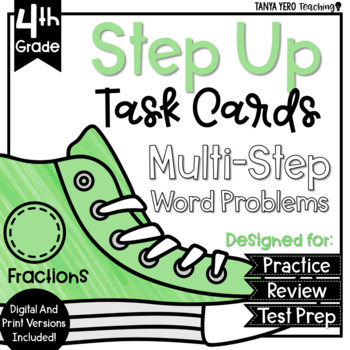# Multi-Step Word Problems 4th Grade Fractions 4.NF.3 - 4.NF.5 GOOGLE CLASSROOM4th - 5th
Subjects
Standards
Resource Type
Formats Included
• PDF
•Google Apps™
Pages
10 pagesThe Teacher-Author indicated this resource includes assets from Google Workspace (e.g. docs, slides, etc.).
##### Also included in
1. This purchase contains 384 task cards that are all multi-step word problems.Build stamina and reduce students’ fear of word problems with Step Up Task Cards! This BUNDLE covers the following standards:1.) 4.NBT.3 - rounding and estimating whole numbers2.) 4.NBT.4 - adding and subtracting multi-digit
Price \$24.00Original Price \$48.00Save \$24.00

### Description

Maximize the versatility of task cards to increase productivity in your classroom.

THIS RESOURCE COMES IN DIGITAL AND PRINT FORMAT. THE DIGITAL VERSION IS COMPATIBLE WITH GOOGLE CLASSROOM/GOOGLE SLIDES!

What are task cards?

Task cards are meant to assess procedural and conceptual understanding of the curriculum you taught to your students. Designed to challenge students, task cards will provide authentic feedback to teachers on student mastery.

How can I use task cards?

➥ Centers/Rotations work

➥ Collaborative group work (Tasks cards make for great discussion starters)

➥ Independent work

➥ Whole group instruction

➥ Interactive Notebook prompts/ questions

This common core aligned purchase contains 24 cards for multi-step word problems which focus on fractions. These word problems are aligned to the following standards: 4.NF.3 -adding and subtracting mixed numbers, 4.NF.4 -apply and extend previous understandings of multiplication to multiply a fraction by a whole number, and 4.NF.5 -express a fraction with denominator 10 as an equivalent fraction with denominator 100, and use this technique to add two fractions with respective denominators 10 and 100. Answer key and recording sheet are included.

Total Pages
10 pages
Included
Teaching Duration
45 minutes
Report this resource to TpT
Reported resources will be reviewed by our team. Report this resource to let us know if this resource violates TpT’s content guidelines.

### Standards

to see state-specific standards (only available in the US).
Understand addition and subtraction of fractions as joining and separating parts referring to the same whole.
Decompose a fraction into a sum of fractions with the same denominator in more than one way, recording each decomposition by an equation. Justify decompositions, e.g., by using a visual fraction model. Examples: 3/8 = 1/8 + 1/8 + 1/8; 3/8 = 1/8 + 2/8; 2 1/8 = 1 + 1 + 1/8 = 8/8 + 8/8 + 1/8.
Add and subtract mixed numbers with like denominators, e.g., by replacing each mixed number with an equivalent fraction, and/or by using properties of operations and the relationship between addition and subtraction.
Solve word problems involving addition and subtraction of fractions referring to the same whole and having like denominators, e.g., by using visual fraction models and equations to represent the problem.
Apply and extend previous understandings of multiplication to multiply a fraction by a whole number.

### Questions & Answers

Teachers Pay Teachers is an online marketplace where teachers buy and sell original educational materials.